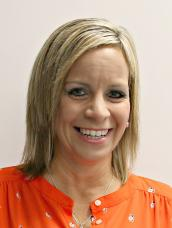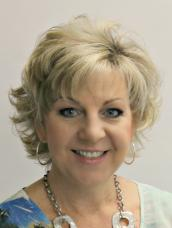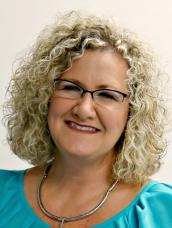# Ashley Long## Ashley Long

Hi... my name is Ashley Long and I am so excited for this school year! I am actually a graduate of McKeel Academy of Technology. After graduating from MAT, I attended the University of South Florida and earned my Bachelors Degree in Elementary Education. I am so blessed to have only ever taught right here at MAC! During my time here at McKeel Academy Central, I have had the pleasure of teaching first, third, and fourth grade.  Two years ago, I was excited to move up and join the sixth grade team. I also teach my favorite subject, math!  As I begin my sixteenth year teaching, my personal philosophy remains the same- no matter the age of the students in my class. I strive everyday to provide a classroom in which every student feels loved, welcome and uplifted- only then can we begin to learn together.

Looking forward to an AMAZING year...

### What We Are Learning

Number Sense & Operations

• Extended knowledge of numbers to negative numbers and develop an understanding of absolute value.
• Add, subtract, multiply, & divide positive rational numbers.
• Apply properties of operations to rewrite numbers in equivalent forms.
• Extend understanding of operations with integers.
• *Add, subtract, multiply, and divide rational numbers.

Algebraic Reasoning

• Apply previous understanding of arithmetic expressions to algebraic expressions.
• Develop an understanding for solving equations and inequalities. Write and solve one-step equations in one variable.
• Understand ratio and unit rate concepts and use them to solve problems.
• *Rewrite algebraic expressions in equivalent form.
• *Write and solve equations and inequalities in one variable.
• *Use percentages and proportional reasoning to solve problems.

Geometric Reasoning

• Apply previous understanding of the coordinate plane to solve problems.
• Model and solve problems involving two-dimensional figures and three-dimensional figures.
• *Solve problems involving two-dimensional figures, including circles.

Data Analysis & Probability

• Develop an understanding of statistics and determine measures of center and measures of variability. Summarize statistical distributions graphically and numerically.
• *Represent and interpret numerical and categorical data.
• *Develop an understanding of probability. Find and compare experimental and theoretical probabilities.

*Accelerated course.

### Important Files#### Kathy Gilbertsen#### Ashley Long#### Deena Masters